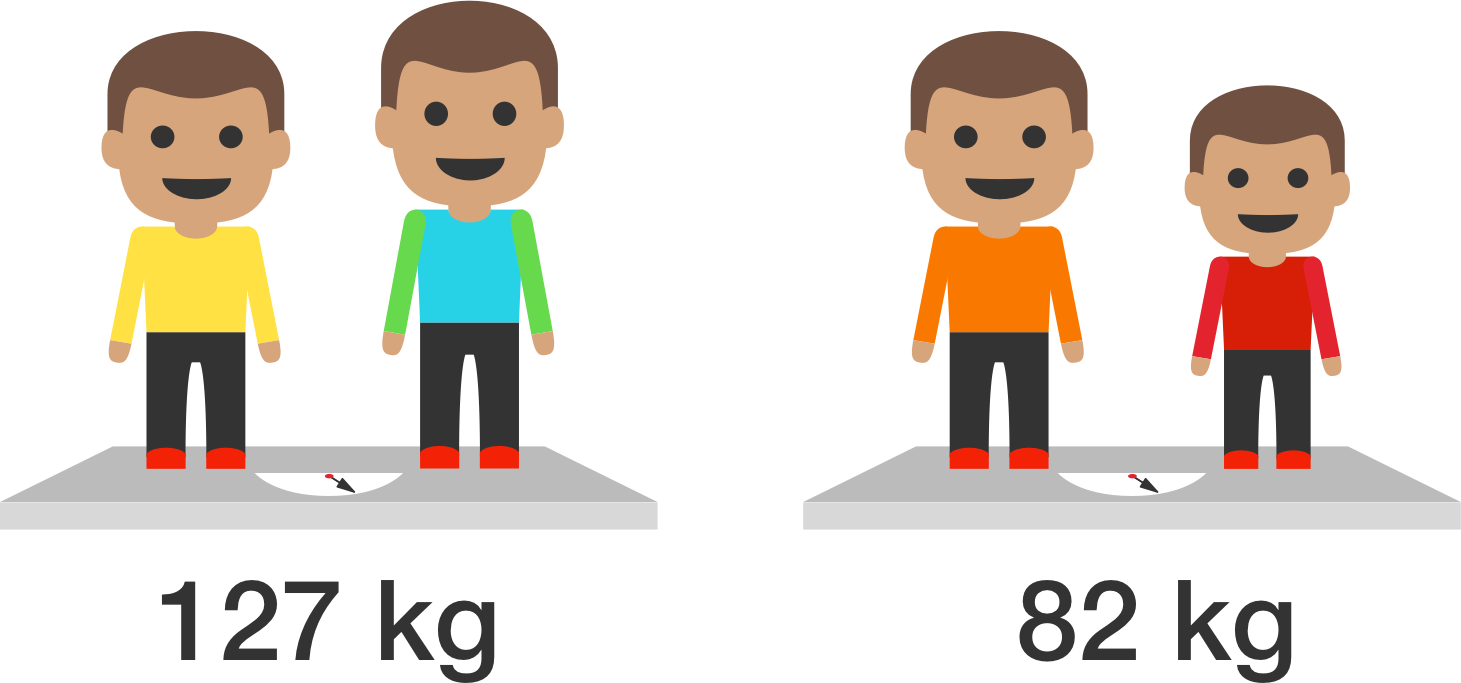# Creative Method To Obtain All The Weights

Algebra Level 3Mr. Gan needs to weigh 5 boys, but his scale can only measure weights of more than $70$ kg.

Since each boy weighed less than $70$ kg, he decided to weigh two boys at a time, making a total of ${ 5 \choose 2} = 10$ weighings.

The readings in kg of the 10 weighings were: $86, 92, 96, 100, 104, 109, 110, 113, 119, 127.$ What was the weight of the second heaviest boy?

×